# Orthoptic (geometry)

In the geometry of curves, an orthoptic is the set of points for which two tangents of a given curve meet at a right angle.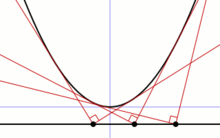The orthoptic of a parabola is its directrix.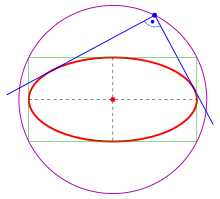Ellipse and its orthoptic (purple)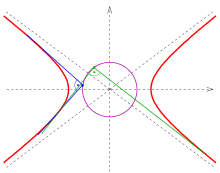Hyperbola with its orthoptic (purple)
Examples

The orthoptic of

1) a parabola is its directrix (proof: s. parabola),
2) an ellipseis the director circle(s. below),
3) a hyperbolais the circle(in case ofthere are no orthogonal tangents, s. below),
4) an astroidis a quadrifolium with the polar equation(s. below).
Generalizations
1) An isoptic is the set of points for which two tangents of a given curve meet at a fixed angle (s. below).
2) An isoptic of two plane curves is the set of points for which two tangents meet at a fixed angle.
3) Thales' theorem on a chordcan be considered as the orthoptic of two circles which are degenerated to the two points.

Remark: In medicine there exists the term orthoptic, too.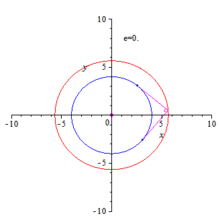Orthoptics (red circles) of a circle, ellipses and hyperbolas

## Orthoptic of an ellipse and hyperbola

### Ellipse

Main article: Director circle

The ellipse with equationcan be represented by the unusual parametric representation

•whereis the slope of the tangent at a point of the ellipse.describes the upper half andthe lower half of the ellipse. The points) with tangents parallel to the y-axis are excluded. But this is no problem, because these tangents meet orthogonal the tangents parallel to the x-axis in the ellipse pointsHence the pointsare points of the desired orthoptic (circle).

The tangent at pointhas the equationIf a tangent contains the point, off the ellipse, then the equationholds. Eliminatig the square root leads towhich has two solutionscorresponding to the two tangents passing point. The free term of a reduced quadratic equation is always the product of its solutions. Hence, if the tangents meet at pointorthogonal, the following equations hold:The last equation is equivalent toThis means:

• The intersection points of orthogonal tangents are points of the circle.

### Hyperbola

The ellipse case can be adopted nearly literally to the hyperbola case. The only changes to be made are: 1) replacebyand 2) restrictby. One gets:

• The intersection points of orthogonal tangents are points of the circle.

## Orthoptic of an astroid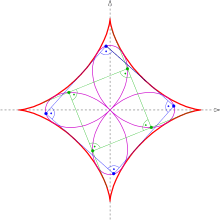Orthoptic (purple) of an astroid

An astroid can be described by the parametric representation.

From the conditionone recognizes, at what distancein parameter space an orthogonal tangent toappears. It turns out, that the distance is independent of parameter, namely. The equations of the (orthogonal) tangents at the pointsandare:.

Their common point has coordinates:,.

This is at the same time a parametric representation of the orthoptic.

Elimination of parameteryields the implicit representationIntroducing the new parameterone gets. (proof uses Angle sum and difference identities.)

Herefrom we get the polar representationof the orthoptic. Hence

## Isoptic of a parabola, an ellipse and a hyperbola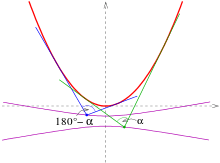Isoptics (purple) of a parabola for angles 80 and 100 degree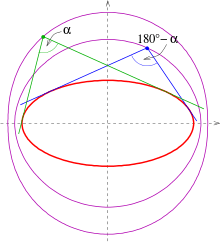Isoptics (purple) of an ellipse for angles 80 and 100 degree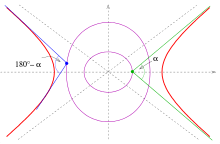Isoptics (purple) of a hyperbola for angles 80 and 100 degree

Below isotopics for an angleare listed. They are called-isoptics. For the proofs: s. below.

### Equations of the isoptics

parabola :

The-isoptics of the parabola with equationare the arms of the hyperbolaThe arms of the hyperbola provide the isoptics for the two angles(s. picture).

Ellipse:

The-isoptics of the ellipse with equationare the two parts of the curve of 4. degree(s. picture).
Hyperbola:

The-isoptics of the hyperbola with the equationare the two parts of the curve of 4. degree### Proofs

Parabola:

A parabolacan be parametrized by the slope of its tangents:The tangent with slopehas the equationPointis on the tangent, ifholds. That means, the slopesof the two tangents containingfulfill the quadratic equationIf the tangents meet with angleor, the equationhas to be fulfilled. Solving the quadratic equation forinsertinginto the last equation, one getsThis is the equation of the hyperbola above. Its arms bear the two isoptics of the parabola for the two anglesand.

Ellipse:

In case of an ellipseone can adopt the idea for the orthoptic until the quadratic equationNow, as in case of a parabola, the quadratic equation has to be solved and the two solutionsto be inserted into the equation. Rearranging shows that the isoptics are parts of the curve of 4. degree:Hyperbola:

The solution for the case of a hyperbola can be adopted from the ellipse case by replacingby(as in the case of the orthoptics, s. above).

Remark: For visualizing the isoptics see implicit curve.Wikimedia Commons has media related to Isoptics.
1. P. K. Jain: Textbook of Analytical Geometry of two Dimensions. p. 214.
• J. Dennis Lawrence (1972). A catalog of special plane curves. Dover Publications. pp. 58–59. ISBN 0-486-60288-5.
• Boris Odehnal: Equioptic Curves of Conic Sections. Journal for Geometry and Graphics, Volume 14 (2010), No. 1, p. 29–43.
• Hermann Schaal: Lineare Algebra und Analytische Geometrie. Band III, Vieweg, 1977, ISBN 3 528 03058 5, p. 220.
• Jacob Steiner’s Vorlesungen über synthetische Geometrie. B. G. Teubner, Leipzig 1867 (bei Google Books), 2. Teil, p. 186.
• Maurizio Ternullo: Two new sets of ellipse related concyclic points. Journal of Geometry 2009, 94, p. 159–173.